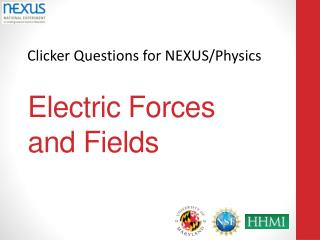DownloadDownload PresentationElectric Forces and Fields

Electric Forces and Fields

Download PresentationElectric Forces and Fields

- - - - - - - - - - - - - - - - - - - - - - - - - - - E N D - - - - - - - - - - - - - - - - - - - - - - - - - - -
Presentation Transcript

1. Electric Forcesand Fields

2. Three identical charges are lined up in a row. If we compare the electric force charge q1exerts on charge q3(F1 →3) to the force q2exerts on charge q3 (F2→3) • F1→3is twice as big as F2→3. • F1→3 is half as big as F2→3. • F1→3is more than twice as big as F2→3. • F1→3is less than half as big as F2→3. • F1→3doesn’t affect q3 at all since q2 is in the way. NEXUS/Physics Clicker Questions

3. In the figure are shown four arrangements of charge. Each charge has the same magnitude, but some are + and some are -. All distances are to the same scale. In which would the magnitude of the force felt by a positive test charge placed at P be the largest? • A • B • C • D • You can’t tell. NEXUS/Physics Clicker Questions

4. In the figure each of the red charges are positive and have the same magnitude. In which of the diagrams would the magnitude of the force felt by a positive test charge placed at P be the largest? • Case 1 • Case 2 • Both are the same • You can’t tell

5. Two small objects each with a net charge of Q(positive) exert a force of magnitude Fon each other. We replace one of the objects with another whose net charge is 4Q. The original magnitude of the force on the Qcharge was F; what is the magnitude of the force on the Q now? • 16F • 4F • F • F/4 • other NEXUS/Physics Clicker Questions

6. In the original state we assumed Qwas positive. If the symbol Qwere taken to have a negative value, how would the forces change compared to the original state? • They would stay the same • Both would reverse • Only the left force would reverse • Only the right force would reverse • Something else NEXUS/Physics Clicker Questions

7. A test charge (labeled q) is placed in a situation in which it feels the electrical force from three other charges (of opposite sign to it) labeled A, B, and C. (The charges are on a uniform grid as shown and the positions are to scale.) Which of the following combinations of forces is the greatest? • There is not enough information to tell. NEXUS/Physics Clicker Questions

8. Two identical conductors hang from nonconducting strings. They are given charges q1 = Q and q2 = 3Q. After charging, the two strings make angles of θ1 and θ2 with the vertical. How do the angles compare? • θ1 > θ2 • θ1 < θ2 • θ1 = θ2 • You don’t have enough informationto tell.

9. In each of the four cases shown below, a particle of charge +qis placed a distance d from a particle of charge +4q. The particles are then released simultaneously. The masses of the particles are indicated in the diagram. Rank the magnitude of the acceleration of the RIGHT HAND particle just after it is released. • Case 1 • Case 2 • Case 3 • Case 4 • Cases 1 & 2 • Cases 1 & 3 • Cases 2 & 4 • Cases 3 & 4 NEXUS/Physics Clicker Questions

10. The electric field at a particular point in space • Depends only on the magnitude of the test charge used to measure it. • Depends only on the sign of the test charge used to measure it. • Depends on both the sign and magnitude of the test charge used to measure it. • Does not depend on the test charge used to measure it. • None of the above. NEXUS/Physics Clicker Questions

11. A test charge, q, is a distance d from a charge Q as shown. It feels an electric field, E0.If qwere replaced by a charge –3q, the electric field it feels would • Change to -3E0 • Change to -E0/3 • Not change • Change to 3E0 • Change to E0/3 • Something else NEXUS/Physics Clicker Questions